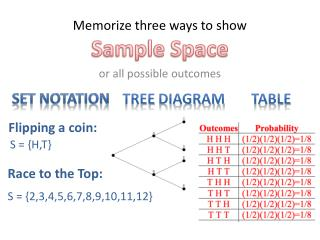# Memorize three ways to show Sample Space - PowerPoint PPT PresentationDownload PresentationMemorize three ways to show Sample Space

Memorize three ways to show Sample Space
Download Presentation## Memorize three ways to show Sample Space

- - - - - - - - - - - - - - - - - - - - - - - - - - - E N D - - - - - - - - - - - - - - - - - - - - - - - - - - -
##### Presentation Transcript

1. or all possible outcomes Memorize three ways to show Sample Space Treediagram Table Flipping a coin: S = {H,T} Set notation Race to the Top: S = {2,3,4,5,6,7,8,9,10,11,12}

2. On a separate piece of paper What is the sample space for the genders in our classroom? What is the sample space for high schools in Iron County? S = {M,F} S = {Canyon View, Cedar, Parowan} 1. Practice Set notation

3. 2. Practice tree diagram Copy the drawing below. Show the sample space of flipping a coin two times H H ½ ½ ½ T H ½ ½ T ½ T

4. 3. Practice table Fill in the table below, showing the 8 possible outcomes of Tossing a coin 3 times.

5. 3. Practice table Fill in the table below, showing the 8 possible outcomes of Tossing a coin 3 times.

6. What are the possible outcomes if you flip a thumbtack?

7. What are the possible outcomes if you flip a tissue box?